Chapter 5: Trusses

# 5.3 Method of Sections

The method of sections uses rigid body analysis to solve for a specific member or two. Instead of looking at each joint, you make a cut through the truss, turning the members along that line into internal forces (assume in tension). Then you solve the rigid body using the equilibrium equations for a rigid body: $\sum F_x=0\;\sum F_y=0\;\sum M_z=0$

The truss:

is split into two to solve for FE.

For this example, you could choose the right half or left half. For some problems, being strategic is necessary otherwise you need to make multiple cuts. In this problem you had to solve for the reaction forces first, but that isn’t always the case as you can sometimes just make the cut (see example 2 below).

Here are more examples of how to make a cut and showing the naming convention: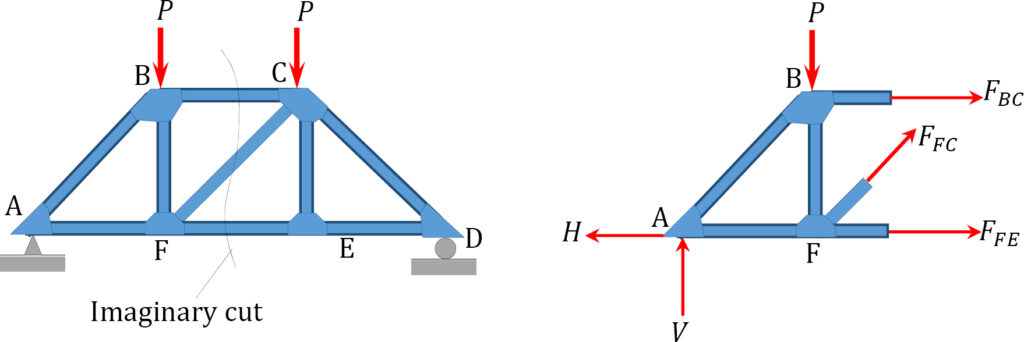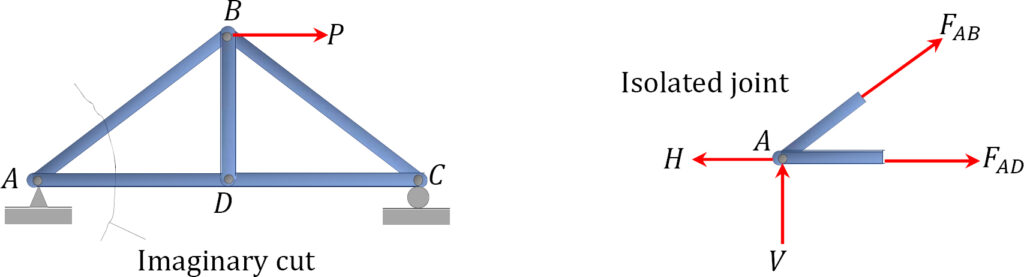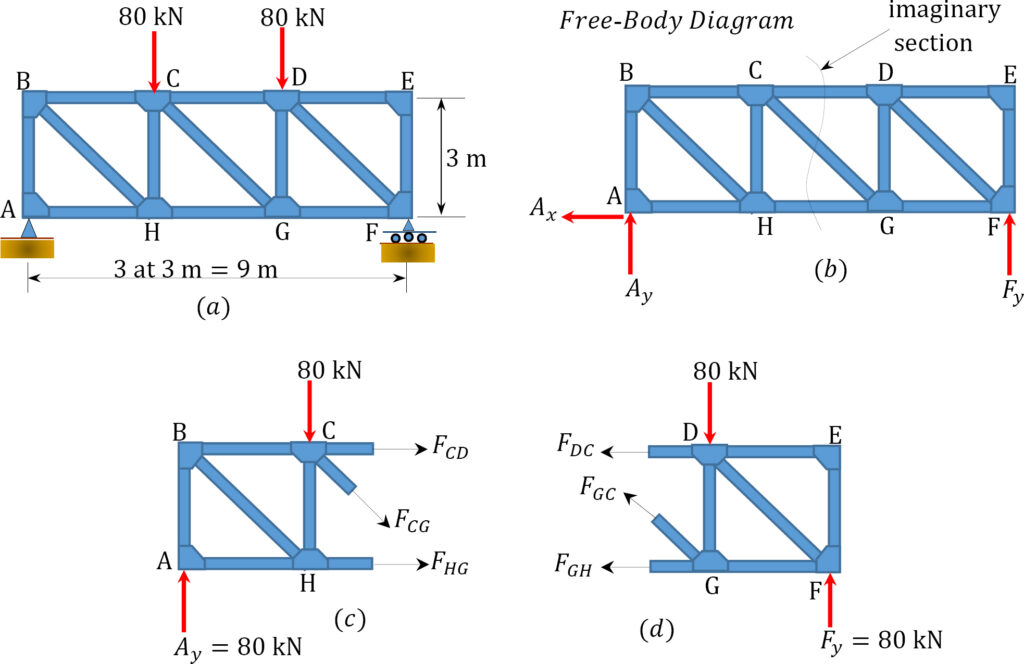Source: Internal Forces in Beams and Frames, Libretexts. https://eng.libretexts.org/Bookshelves/Civil_Engineering/Book%3A_Structural_Analysis_(Udoeyo)/01%3A_Chapters/1.05%3A_Internal_Forces_in_Plane_Trusses

Here is a detailed explanation:

The method of sections is a process used to solve for the unknown forces acting on members of a truss. The method involves breaking the truss down into individual sections and analyzing each section as a separate rigid body. The method of sections is usually the fastest and easiest way to determine the unknown forces acting in a specific member of the truss.

## Using This Method:

The process used in the method of sections is outlined below:

1. In the beginning it is usually useful to label the members in your truss. This will help you keep everything organized and consistent in later analysis. In this book, the members will be labeled with letters.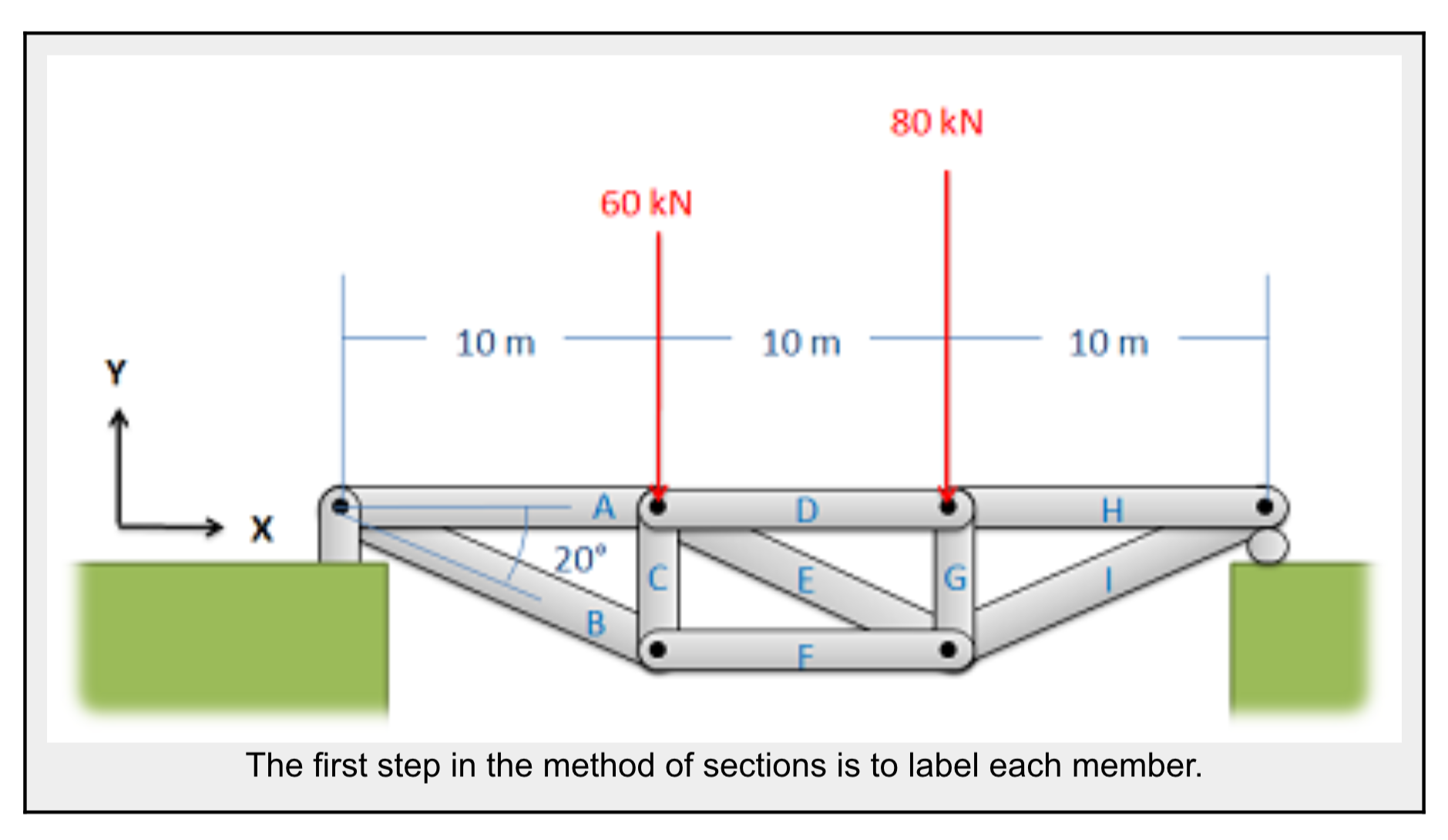2. Treating the entire truss structure as a rigid body, draw a free body diagram, write out the equilibrium equations, and solve for the external reacting forces acting on the truss structure. This analysis should not differ from the analysis of a single rigid body.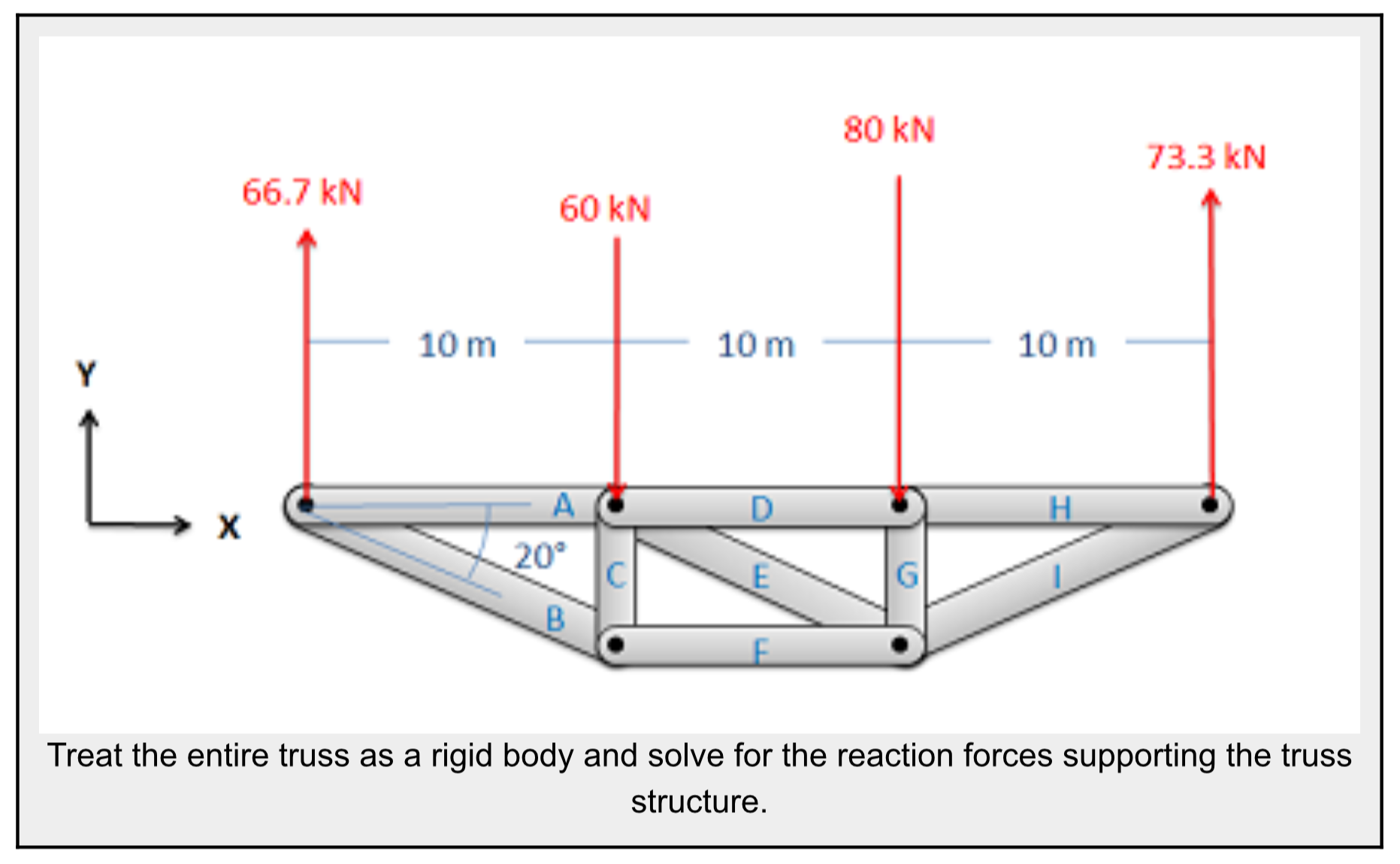3. Next you will imagine cutting your truss into two separate sections. The cut should travel through the member that you are trying to solve for the forces in, and should cut through as few members as possible (The cut does not need to be a straight line).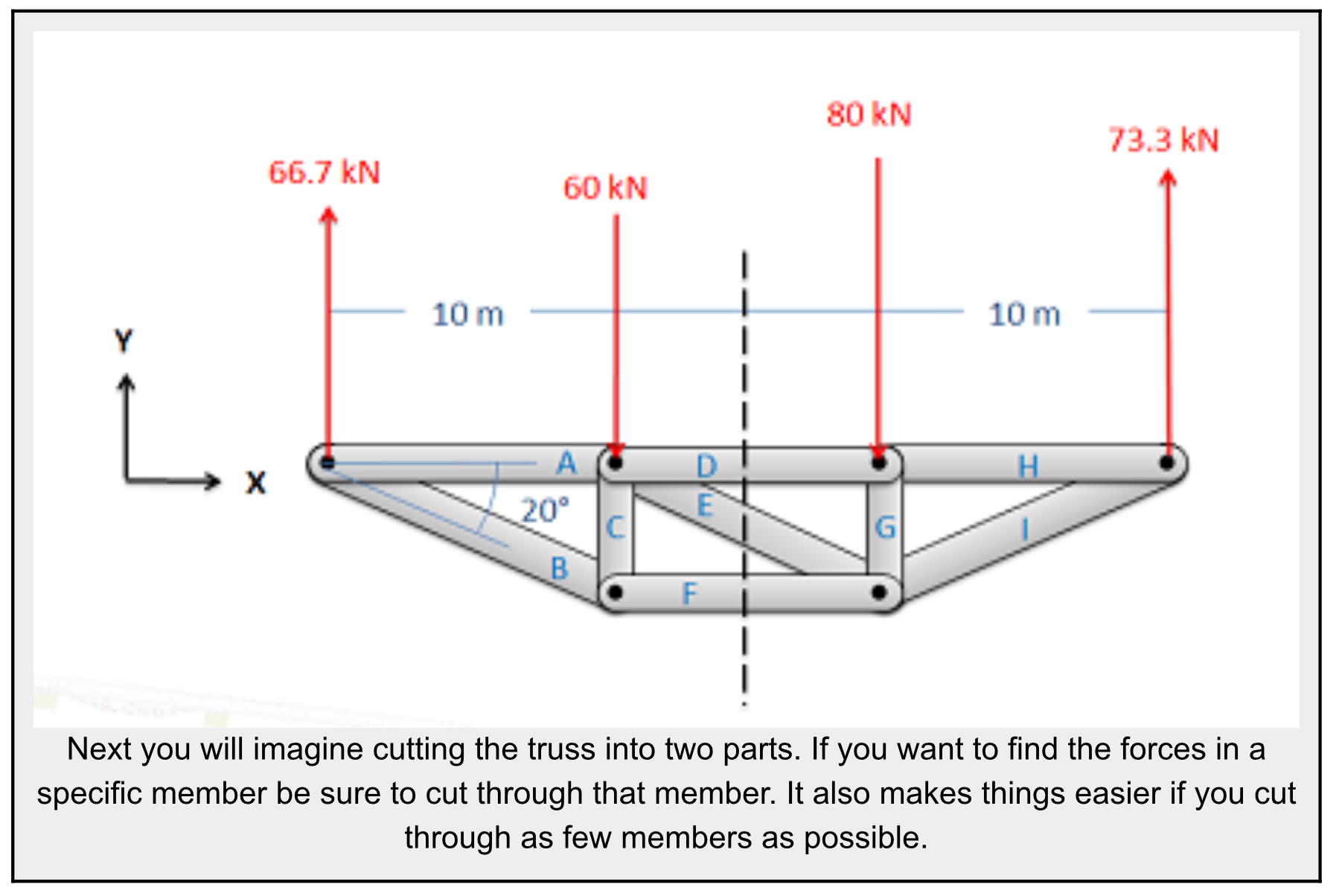4. Next you will draw a free body diagram for either one, or both sections that you created. Be sure to include all the forces acting on each section.
• Any external reaction or load forces that may be acting at the section.
• An internal force in each member that was cut when splitting the truss into sections. Remember that for a two force member, the force will be acting along the line between the two connection points on the member. We will also need to guess if it will be a tensile or a compressive force. An incorrect guess now though will simply lead to a negative solution later on. A common strategy then is to assume all forces are tensile, then later in the solution any positive forces will be tensile forces and any negative forces will be compressive forces.
• Label each force in the diagram. Include any known magnitudes and directions and provide variable names for each unknown.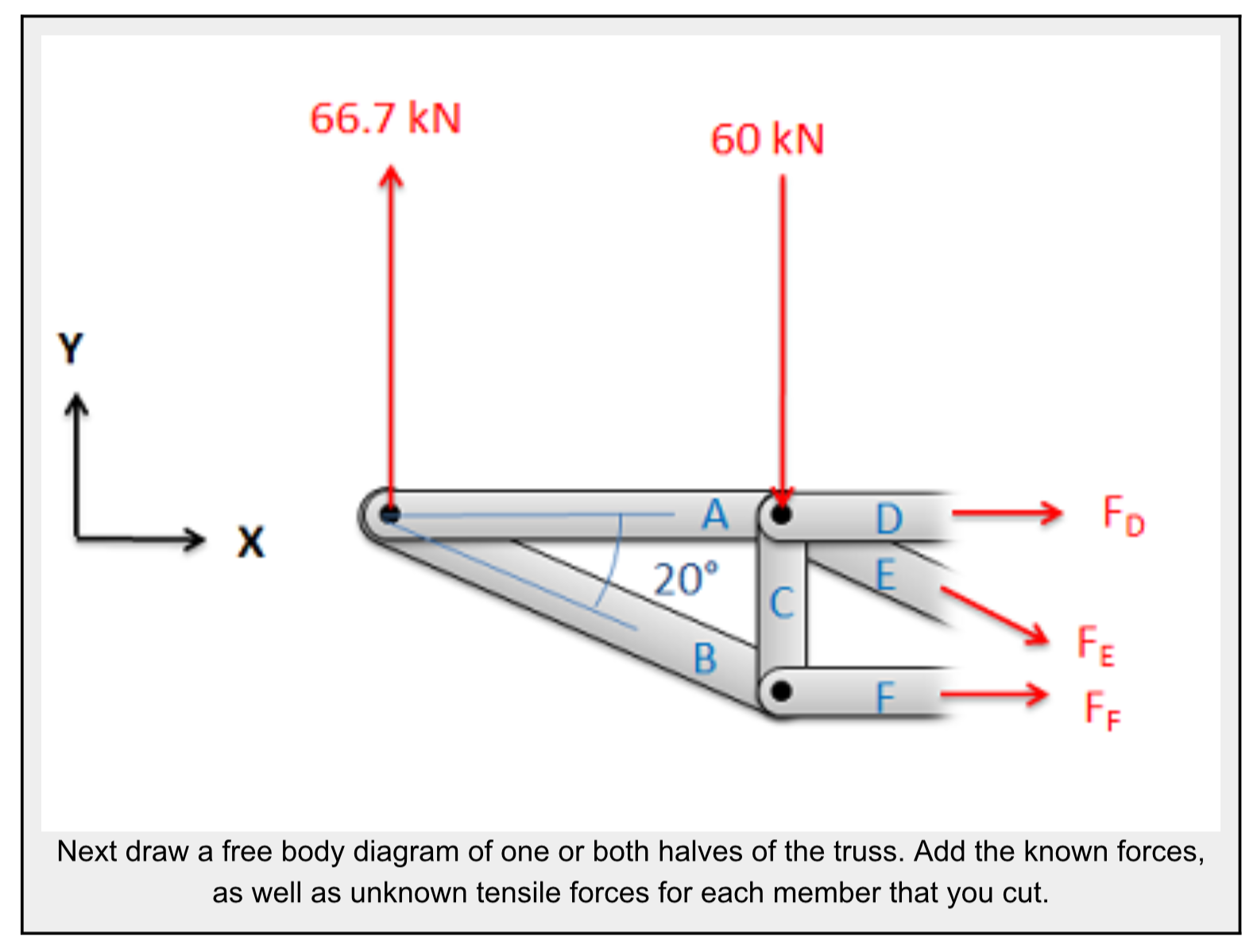5. Write out the equilibrium equations for each section you drew a free body diagram of. These will be extended bodies, so you will need to write out the force and the moment equations.
• You will have three possible equations for each section, two force equations and one moment equation.$$\sum\vec F=0\; \; \sum\vec M=0\\\sum F_x=0\; \; \sum F_y=0\; \; \sum M_z=0$$
6. Finally, solve the equilibrium equations for the unknowns. You can do this algebraically, solving for one variable at a time, or you can use matrix equations to solve for everything at once. If you assumed that all forces were tensile earlier, remember that negative answers indicate compressive forces in the members.

Source:Engineering Mechanics, Jacob Moore, et al. http://mechanicsmap.psu.edu/websites/5_structures/5-5_method_of_sections/methodofsections.html

Additional examples from the Engineering Mechanics webpage:

#### Example 1:

Find the forces acting on members BD and CE. Be sure to indicate if the forces are tensile or compressive.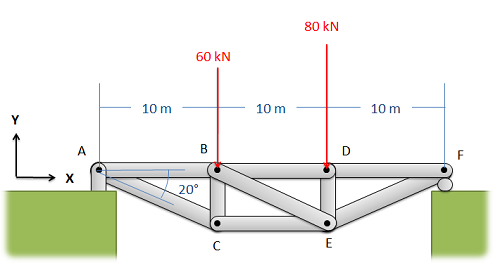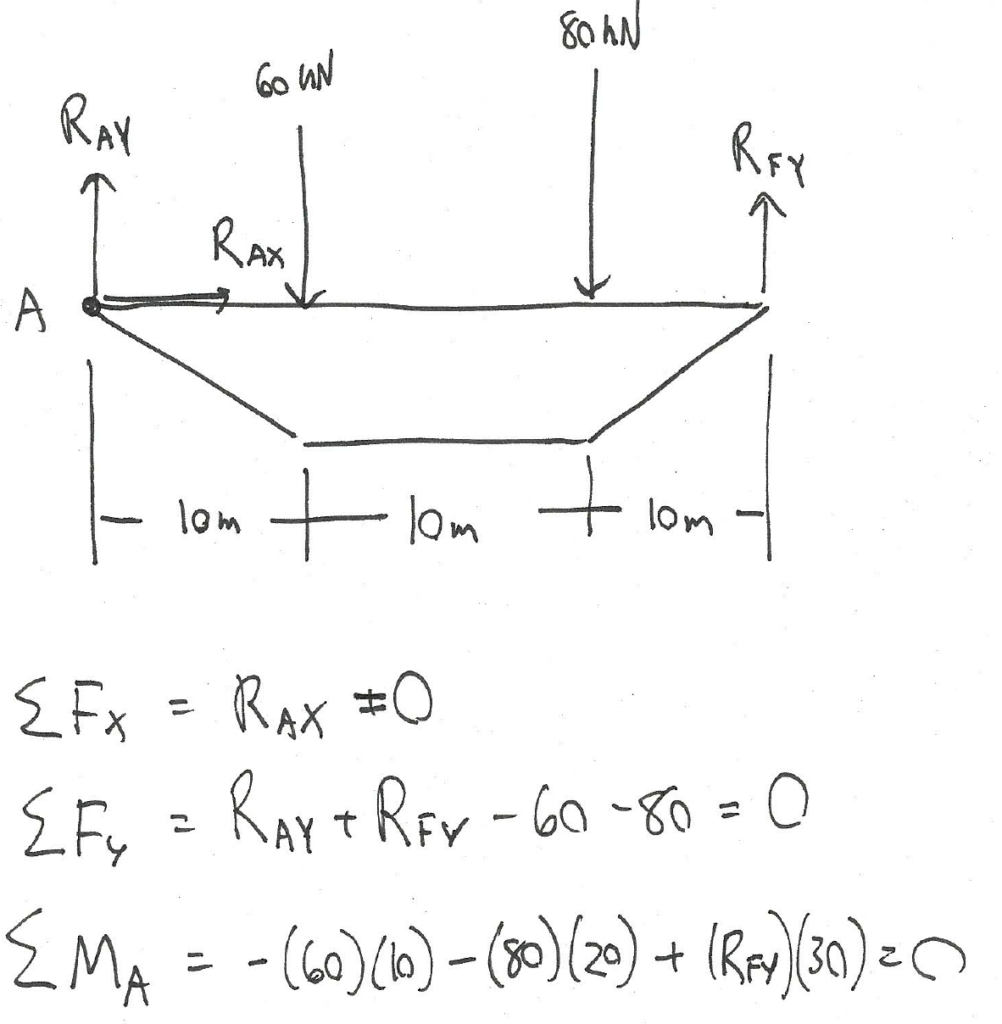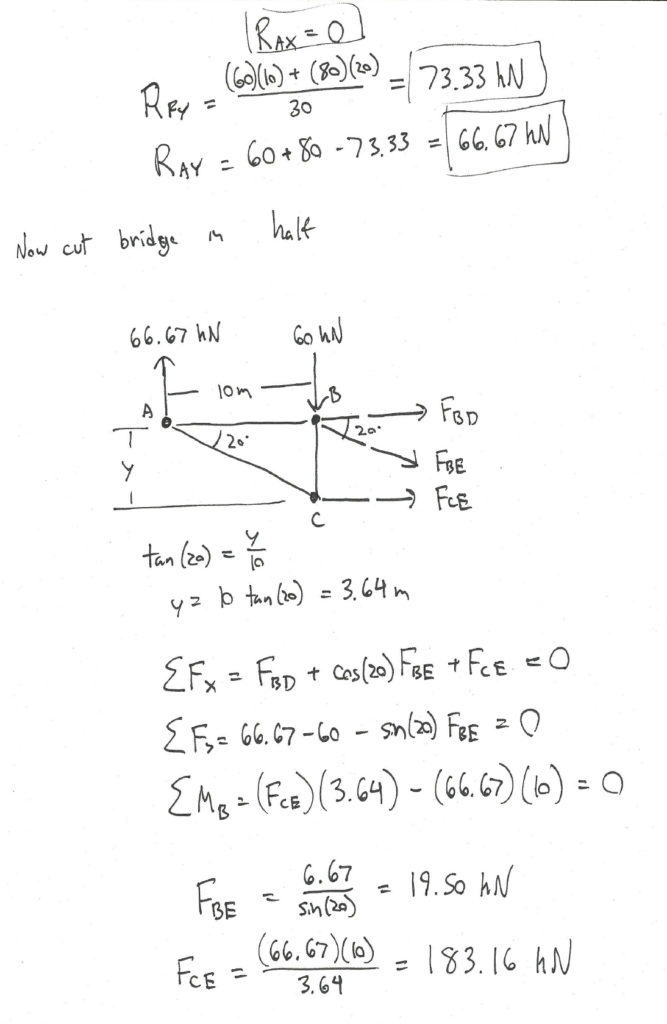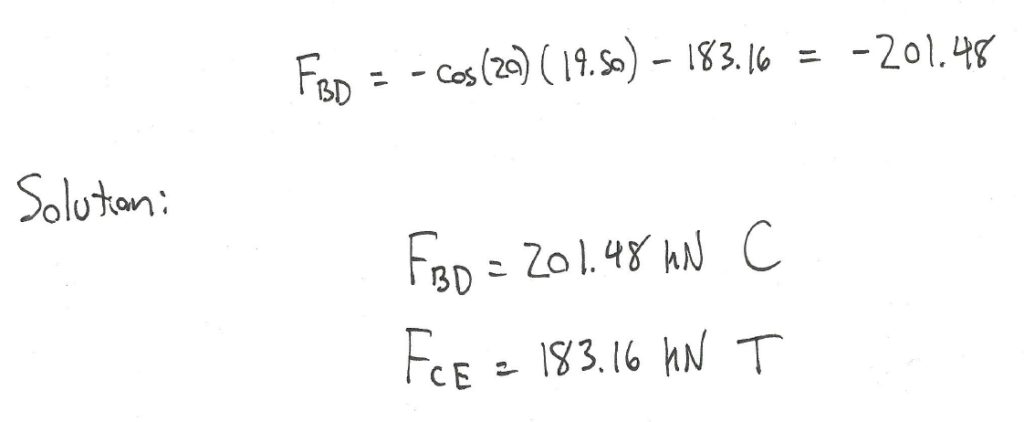Source: Engineering Mechanics, Jacob Moore, et al. http://mechanicsmap.psu.edu/websites/5_structures/5-5_method_of_sections/pdf/MethodOfSections_WorkedExample1.pdf

#### Example 2:

Find the forces acting on members AC, BC, and BD of the truss. Be sure to indicate if the forces are tensile or compressive.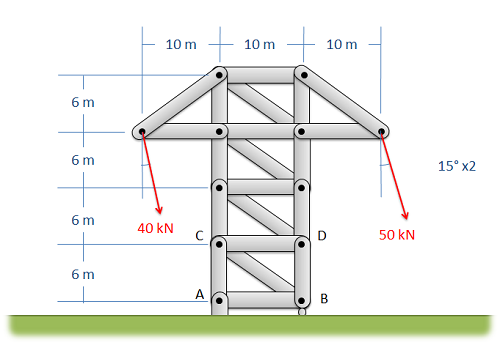If we make a cut in the top section, we don’t need to solve for the reaction forces.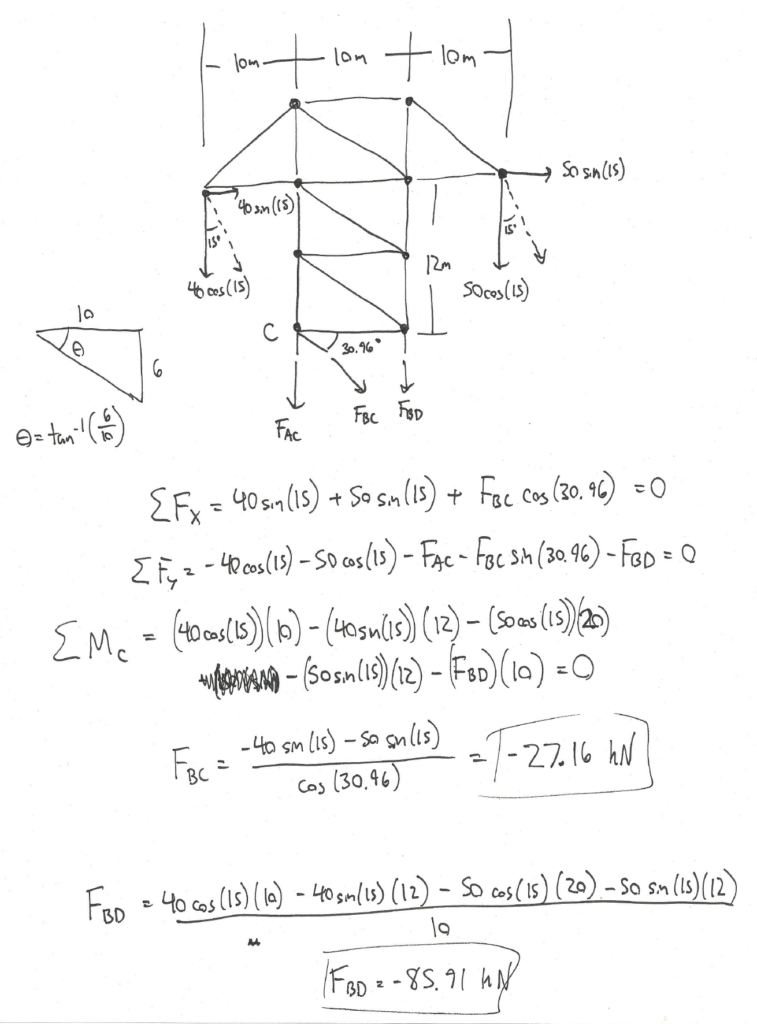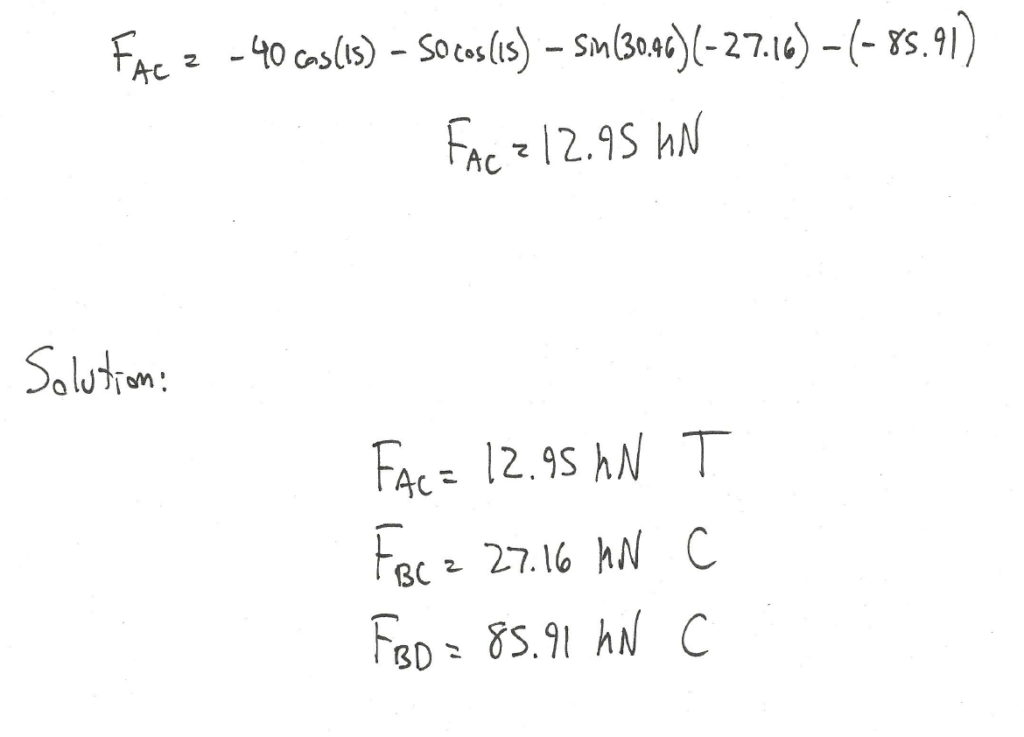Source: Engineering Mechanics, Jacob Moore, et al.  http://mechanicsmap.psu.edu/websites/5_structures/5-5_method_of_sections/pdf/MethodOfSections_WorkedExample2.pdf

In summary: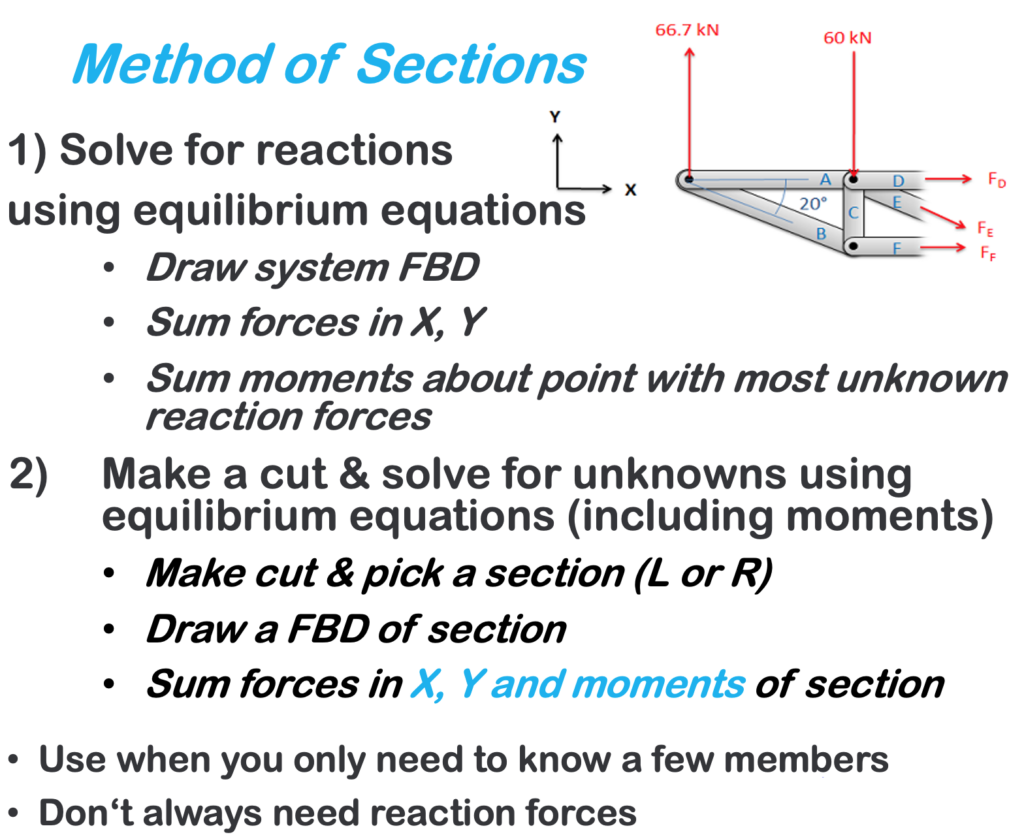Key Takeaways

Basically: Method of sections is an analysis technique to find the forces in some members of a truss. It separates the truss into two sections then uses the rigid body equilibrium equations.

Application: To calculate the loads on bridges and roofs, especially if you need to know only one or two of the members.

Looking Ahead: The next section explores a trick that makes solving faster, especially for method of joints.